MATH125: Unit 1 Individual ProjectAnswer Form Mathematical Modeling and Problem Solving

MATH125: Unit 1 Individual ProjectAnswer Form

Mathematical Modeling and Problem Solving

ALL questions below regarding SENDING A PACKAGE and PAINTING A BEDROOM must be answered. Show ALL step-by-step calculations, round all of your final answers correctly, and include the units of measurement. Submit this modifiedAnswer Formin the Unit 1 IP Submissions area.

All commonly used formulas for geometric objects are really mathematical models of the characteristics of physical objects. For example, a basketball, because it is a sphere, can be partially modeled by its distance from one side through the center (radius, r) and then to the other sideby the diameter formula for a sphere:D = 2r.

For familiar two-dimensional variables length, L, and width, W, the perimeter and area formulas for a rectangle are mathematical models for distance around the rectangle (perimeter, P) and the region enclosed by the sides (area, A), respectively:

P = 2L + 2W and A = L x W

Along with another variable, height,H, a three-dimensional rectangular prism’s volume and surface area can be measured. For example, the formulas for a common closed cardboard box’s inside space (volume, V) and outside covering (surface area, SA) are respectively:

V = L x W x H and SA = 2(L x W) + 2(W x H) + 2(L x H)

For this IP assignment follow Polya’s principles to solve your chosen problem, and include the following:

·         Develop and write down a strategy for solving this problem; show the steps in the correct order for your attempted solution.

·         Did your strategy actually solve the problem? How do you know?

·         Suppose your solution did not solve the problemwhat would be your next action?

SENDING A PACKAGE

Your goal is to construct a rectangular box with a top on it that has the smallest possible surface area in which a football and a basketball, both fully inflated, will just fit into at the same time.The following are the measurements of the football and basketball: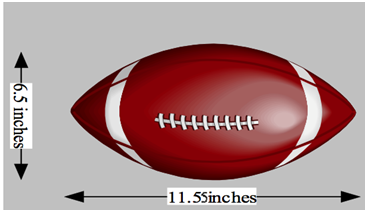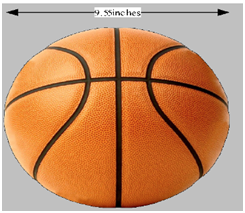1.       What box dimensions make a good model for this situation? All quantities are inside-of-the-box measurements.First, position the football and basketball side-by-side. Then, slide the basketball so that it is even with one point of the football. Now, measurements can be made that will give the minimum width across both objects. That will be the minimum width of the box with the smallest surface area. Using the following diagrams, first find the exact LENGTH and HEIGHT: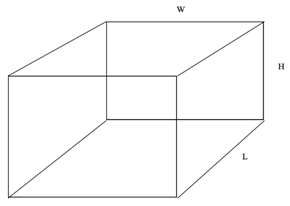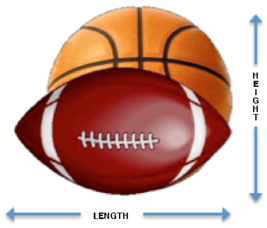1.       Note that the diameters combined include an overlap; see t

Here is the right triangle shown larger and labeled:

he cross-section perspective below. To find the WIDTH, you must first account for this by applying the Pythagorean theorem. The WIDTH will be the radius of the football plus the side b of the right triangle below plus the radius of the basketball.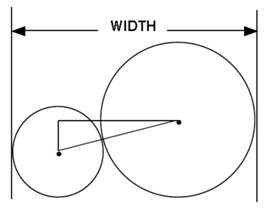Here is the right triangle shown larger and labeled: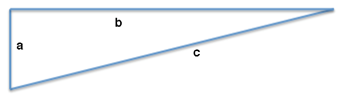Find a and c. The measure of the hypotenuse, c, is the sum of the two balls’ radii. The smaller side, a, is the difference of these two radii. Find these two exact sidesincluding the units of measurement:

1.       Next, find b.Apply the Pythagorean theorem, using itsform:Show all step-by-step calculations, including the units of measurement, and round your final answer to the nearest hundredth:

2.       Now, list all of the box’s dimensions in the chart below.Recallfrom above: The WIDTH will be the radius of the football plus the radius of the basketball plus the side b of the right triangle above.

Show all step-by-step calculations, including the units of measurement, and round your final answers to the nearest tenth:

3.       Using Polya’s technique for solving problems, describe and discuss the strategy, steps, formulas, and procedures you will use to solve this problem.

4.       The minimum surface area corresponds to the minimum volume. Using the formula and dimensions from above, find the box’s volume.

Show all step-by-step calculations, including the units of measurement, and round your final answers to the nearest whole measurement unit:

5.       Using the formulaand dimensions from above, find the box’s surface area.

Show all step-by-step calculations, including the units of measurement, and round your final answers to the nearest whole measurement unit:

6.       Demonstrate that your solution is correct. In other words, explain why the box you have created is the smallest possible box.

PAINTING A BEDROOM

The walls and ceiling in your bedroom need to be painted, and the painters’ estimates to do the work are far too expensive. You decide that you will paint the bedroom yourself. Below is the information to help you solve the problem:

·         The bedroom is 17 ft.,3 in. long by 18 ft. wide, and the ceiling is 9 ft. high.

·         The color of paint you have selected for the walls covers 84 square feet per gallon and costs \$31.50 per gallon.

·         The inside of the bedroom door is to be painted the same color as the walls.

·         The ceiling will be painted with a bright white ceiling paint that costs \$27.50 per gallon but only covers 73 sq. ft. per gallon.

·         Two coats of paint will be applied to all painted surfaces.

·         The room has one window, measuring 3 ft.,3 in. by 4 ft., which will not be painted.

1.       Because different paint lots of the same color may appear slightlydifferent in color, when painting a room, you should buy all of your paint at one time and intermixthe paint from at least two different cans so that the walls will all be exactly the same color.Because all ending values are given in feet, first find the room dimensions in feet that make a good model for this situation.ANSWERS LENGTH ft. WIDTH ft. HEIGHT ft.

2.       Using the measurements found above, label the sides in feet here:

Front wall and back wall:

Left wall and right wall:

Ceiling:

Window:

 Diagram 2 Wall 14.25’ by 8’

3.       Using the formula conceptsand dimensions from above, find the bedroom’s total painted surface area around all of the walls, including both coats. Do not forget to subtract the window’s area. Also, double the paint to account for two coats.

Show all step-by-step calculations, including the units of measurement, and round your final answers to the nearest whole measurement unit:

 ANSWER Total painted wall surface area

4.       Using the formula conceptsand dimensions from above, find the ceiling’s total painted surface area, including both coats.

Show all step-by-step calculations, including the units of measurement, and round your final answers to the nearest whole measurement unit:

 ANSWER Total painted ceiling surface area

5.       Describe and discuss the strategy, steps, formulas, and procedures for how you will use Polya’s problem-solving techniques to determine how much it will cost to paint this bedroom with two coats of paint (on all walls and the ceiling).

6.       Find,individually and as a total, how much it will cost to paint this bedroom with two coats of paint (on all walls and the ceiling).

Show all step-by-step calculations, including the units of measurement, and round your final answers to the nearest whole dollar amount:

 ANSWERS Total cost painted wall surface area Total cost ceiling surface area Overall total cost of paint

7.       Assuming you can paint 100 sq. ft. per hour, what will be the work time needed to paint your bedroom?

Show all step-by-step calculations, including the units of measurement, and round your final answers to the nearest whole hour amount:

Field of study: